Search
 Submission Procedure share: |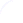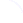available in:   PDF (211 kB) PS (58 kB)

get:
 Similar Docs BibTeX Write a comment
get:

DOI:   10.3217/jucs-004-01-0068

### Componentwise Distance to Singularity

G. Rex (Institute of Mathematics, University of Leipzig, Germany)

Abstract:

A perturbation matrix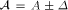is considered, where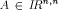and. The matrix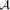is singular iffcontains a real singular matrix. A problem is to decide ifis singular or nonsingular, a NP-hard problem. The decision can be made by the computation of the componentwise distance to the nearest singular matrix defined on the basis of the real spectral radius, and by the solution of 4n eigenvalue problems.

Theorem 6 gives a new computation basis, a natural way to the "componentwise distance ..." definition, and a motivation to rename this in radius of singularity denoted by sir.

This new way shows: (i) - sir results from a real nonnegative eigensolution of a non-linear mapping, (ii) - sir has a norm representation, (iii) - sir can be computed by 2n-1 nonnegative eigensolutions of the nonlinear mapping, (iv) - for the special case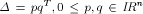a formula for a computation of sir is given, also a trivial algorithm for the computation, and some examples as demonstration.

Keywords: NP-hard, componentwise distance to the nearest singular matrix, interval matrix, perturbation matrix, radius of singularity